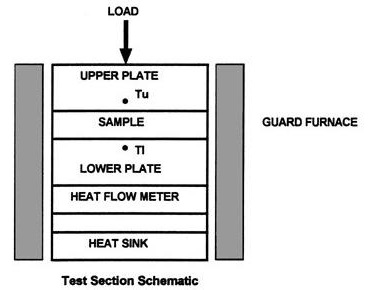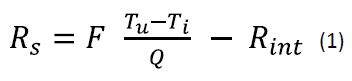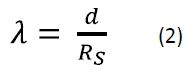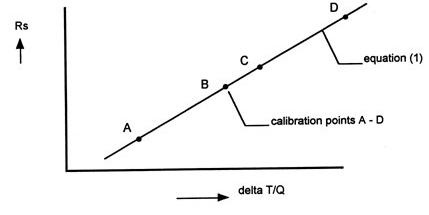# Measuring Thermal Conductivity According to the ASTM E1530 Guarded Heat Flow Meter Method

TA Instruments has introduced a range of instruments for the accurate measurement of heat transfer properties in a wide range of materials. The DTC-25 and DTC-300 thermal conductvitity meters provide direct measurement of thermal conductivity based on the ASTM E1530 guarded heat flow meter method.

The DTC-300 meter consists of a guard furnace surrounding the test stack to mitigate the heat transfer effects along the sample edges thereby avoiding measurement errors. The DTC-25 meter, however, operates only at room temperature without requiring a guard furnace.

## Working Principle of DTC Instruments

A sample material to be tested is placed in a compressive load between two polished metal surfaces of varying temperatures. The lower polished surface is part of a calibrated heat flux transducer. An axial temperature gradient is generated in the pile with the flow of heat from the upper surface to the lower surface via the sample. The thermal conductivity of the sample can be evaluated using the measure of temperature difference of the sample along with the heat flux transducer output. Figure 1 shows the schematic of test section of DTC instruments.Figure 1. Schematic of DTC Test Section

## Analysis of Guarded Heat Flow Meter Data

The Fourier heat flow equation applied to the test section at thermal equilibrium is given by the Equation 1.where

Rint = interface thermal resistance

Q = heat flow transducer output

TI = lower plate surface temperature

Tu = upper plate surface temperature

F = heat flow transducer calibration factor

Rs = thermal resistance of the sample

The sample thermal conductivity (λ) can be determined using the Equation 2.where

d = sample thickness

Both Rint and F in Equation 1 are obtained during calibration of the equipment. Equation 1 displays a linear relationship between DT/Q and Rs. It can be plotted on a graph as a straight line with axis intersection at –Rint and slope F. The corresponding values of DT/Q can be obtained by testing samples of known thermal conductivity and known Rs value. The straight line obtained using the data points can be used for subsequent testing of unknown samples. Figure 2 shows the analysis of guarded heat flow meter data.Figure 2. Guarded heat flow meter data analysis

By reducing the value of Rint , it is possible to obtain high test accuracy. In order to achieve this, highly polished metal surfaces are made to contact with the sample by clamping the sample using a reproducible force or by placing heat transfer compound on the contact surfaces. A pneumatic cylinder can be used to produce the axial clamping force in the DTC instruments. The pressure of reproducible air is controlled by a pressure regulator.

The DTC-300 instrument needs to be calibrated at different temperatures to provide a set of linear equations as Equation (1) is slightly temperature dependent. Linear interpolation is allowed while testing at sample temperatures as the coefficients of Equation (1) do not vary much on the temperature range of the instrument. TA Instruments has developed software for a PC to perform the data analysis. Using this software, the thermal conductivity of the sample is automatically computed upon entering the data obtained from the digital display of the instrument.This information has been sourced, reviewed and adapted from materials provided by TA Instruments.

## Citations

• APA

TA Instruments. (2019, July 31). Measuring Thermal Conductivity According to the ASTM E1530 Guarded Heat Flow Meter Method. AZoM. Retrieved on February 19, 2020 from https://www.azom.com/article.aspx?ArticleID=11518.

• MLA

TA Instruments. "Measuring Thermal Conductivity According to the ASTM E1530 Guarded Heat Flow Meter Method". AZoM. 19 February 2020. <https://www.azom.com/article.aspx?ArticleID=11518>.

• Chicago

TA Instruments. "Measuring Thermal Conductivity According to the ASTM E1530 Guarded Heat Flow Meter Method". AZoM. https://www.azom.com/article.aspx?ArticleID=11518. (accessed February 19, 2020).

• Harvard

TA Instruments. 2019. Measuring Thermal Conductivity According to the ASTM E1530 Guarded Heat Flow Meter Method. AZoM, viewed 19 February 2020, https://www.azom.com/article.aspx?ArticleID=11518.

1.l msays:

Hi!
Just wondering for the calibration part, how do we get the right DT/Q that matches a known Rs more precisely?
Thanks

The opinions expressed here are the views of the writer and do not necessarily reflect the views and opinions of AZoM.com.